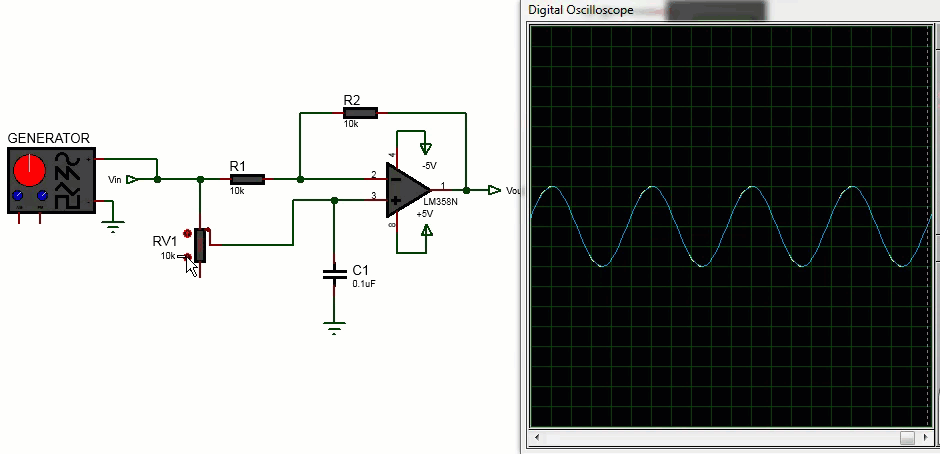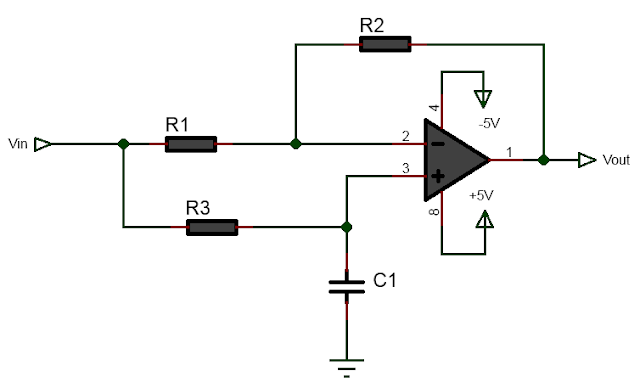# what is phase shifter circuit and how to build it?

A phase shifter circuit is an electronic circuit that is used to shift the phase of a signal without changing its amplitude. It is a fundamental component in many signal processing systems and is used in applications such as audio processing, frequency synthesis, and phase modulation, AM modulation and demodulation, SSB modulation.There are several ways to build a phase shifter circuit, but one common approach is to use a resistor-capacitor (RC) lag network connected to the non-inverting terminal of an op-amp. In this configuration, the phase shift is determined by the ratio of the input signal frequency and the cutoff frequency of the RC lag network, that is, resistance to the capacitance in the network. The op-amp is connected in inverting configuration with unity gain, that is equal resistors on the inverting input. The circuit can ideally shift phase of an input signal from 0 degree to 180 degree without changing the amplitude of the signal. In this article it is explained how an op-amp based phase shifter circuit works and how to build it. Also included is online phase difference calculator for calculating the resistor value given the input frequency and capacitor for desired phase difference.

Here's how to build a basic op-amp based RC phase shifter circuit:

Materials Required:

• op-amp(LM358, LM324, TL072 or similar)
• two equal resistors
• variable resistor
• one capacitor
• jumper wires
• power supply

Following shows the circuit diagram of phase shifter circuit implemented using operational amplifier. Build the circuit on breadboard following the circuit diagram.

How Op-Amp Phase Shifter Circuit works

In the above circuit, the inverting input two equal resistors R1 and R2 are connected.  The non-inverting input is connected to R3C1 lag circuit.

The inverting circuit has two equal resistors and so the inverting voltage gain is always 1.

$$A_v(invert) = -\frac{R2}{R1} = -1$$   -------->(1)

However the gain from the non-inverting input depends upon the cutoff frequency of the R3C1 lag circuit.

$$f_c = \frac{1}{2 \pi R_3 C_1}$$   -------->(2)

Consider the following two cases

(a) when fi >>fc

(b) when fi<<fc

(a) when fi >>fc

When the frequency(fi) of the input signal is much greater than the cutoff frequency of the R3C1 lag circuit then the capacitor appear shorted to ground. This is because the reactance of C1 is very low, Xc=1/2*pi*fi*C at high frequency fi. Because the capacitor is shorted, the input to the non-inverting terminal is grounded, the input is zero and the non-inverting voltage gain is zero. So at much higher frequency than the cutoff frequency of the filter, the output signal is due to the inverting terminal only and the overall gain is -1, that is the output signal is phase shifted by 180 degree.

So mathematically in this case we write

$$A_v = A_v(invert) +A_v(non-invert)$$   -------->(3)

where Av is the overall gain of the op-amp

or, $$A_v = -1 + 0 = -1$$

(b) when fi<<fc

When the input signal frequency much less than the cutoff frequency the R3C1 lag network, the capacitor C1 appears open. Since C1 is open circuit, the input signal goes into both the inverting as well as the non-inverting terminal. And in this case the total voltage gain is calculated as follows.

$$A_v = A_v(invert) +A_v(non-invert)$$

or,  $$A_v = -1 +A_v(non-invert)$$

But, $$A_v(non-invert) = 1+\frac{R2}{R3}=1+1=2$$ when R2=R3

that is, $$A_v = -1 +2=1$$

So when R2=R3, the total voltage gain unity which means that the output signal has the same amplitude as that of input signal and that the phase difference is 0.

Phase Difference Angle

The phase difference angle is given by the following equation,

$$\tan(\frac{\phi}{2})=\frac{f_i}{f_c}$$  -------->(4)

where fc is the cutoff frequency of R3C1 network and fi is the input signal frequency.

Since we have,

$$f_c = \frac{1}{2 \pi R_3 C_1}$$

Substituting fc in equation(4), we get

$$\tan(\frac{\phi}{2})=2 \pi f_i R_3 C_1$$

Solving for R3

$$R_3=\frac{\tan(\frac{\phi}{2})}{2 \pi f_i C_1}$$   -------->(5)

Picture below illustrates phase difference between the input and output signal.

Online Phase Shifter Circuit Calculator

Using equation(5) the following is calculator that calculates resistor R3 from desired phase difference, given capacitor C1 and the input frequency.

Result:Example calculation:

Let us suppose that we want to build 90 degree phase shifter circuit. The resistors R1 and R2 can be chosen arbitrarily, say 10KOhm. Let the input frequency be fi=1KHz, then we can use the above equation or the calculator to calculate the required value of resistor R3 given C1. Let C1=0.1uF. Then we have,

$$R_3=\frac{\tan(\frac{\phi}{2})}{2 \pi f_i C_1}$$

or,  $$R_3=\frac{\tan(\frac{90}{2})}{2 \times 3.14 \times 1KHz \times 0.1\mu F} = 1.59k\Omega$$

Following figure shows the 90 degree phase shifter circuit with the calculated values.

Similarly for 180 phase shifter, the value of R3 is 912.35KOhm.

Video demonstration

The following video demonstrates how the op-amp based phase shifter circuit works. To control the phase difference between the input and output signal a 10KOhm potentiometer is used for illustration purpose.

Conclusion

Phase shifter circuit is required in number of electronics circuit such as in communication electronics circuits, digital analog signal processing, analog signal processing etc. Phase shifter can be build with an operational amplifier connected such that it works on inverting configuration as well as both inverting and non-inverting configuration. A RC lag network is connected to the non-inverting op-amp such as LM358N op-amp or LM324 op-amp or similar op-amp. The working principle of the phase shifter circuit can be understood by considering what happens when the input signal frequency is higher or lower compared to the cutoff frequency of the RC network. When the frequency is higher than the cutoff frequency of RC lag network, the non-inverting terminal is grounded and so the phase shifter acts as inverting amplifier with unity gain. In this case the output signal is equal in amplitude to the input signal but there is 180 phase difference between them. When the input signal frequency is greater than the cutoff frequency then the capacitor is open circuit and the input signal is applied to both the inverting and non-inverting terminal and so the output signal is equal in both the magnitude and phase compared to the input signal. In between we can change the cutoff frequency by varying either capacitor or resistor of the RC network to change the cutoff frequency to change the phase difference between the input and output signal. The amplitude however remains unchanged. The phase difference is given by the ratio of the input signal frequency and the RC network cutoff frequency.

References: